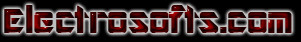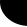HOME Electronics Directory Articles/ Tutorials eBooks About Us FORUM Links Contact UsVerilog Tutorial: Harsha Perla

Verilog Loop Statements

Loop statements are used to control repeated execution of one or more statements. There are 4 types of looping stetements in Verilog:

forever statement;

repeat(expression) statement;

while(expression) statement;

for(initial_assignment; expression; step_assignment) statement;

We can combine more than one statements using begin -- end block in an looping instruction. Looping statements should be used within a procedural block.

forever Loop:

The forever instruction continuously repeats the statement that follows it. Therefore, it should be used with procedural timing controls (otherwise it hangs the simulation). Consider this example:

```initial
begin
clk = 0;
forever #5 clk = ~clk;
end ```
```repeat Loop:
```

Repeats the following instruction for specified times. The number of executions is set by the expression or constant value. If expression evaluates to high impedance or un-known, then statement will not be executed.

```initial
begin
x = 0;
repeat( 16 )
begin
#2 \$display("y= ", y);
x = x + 1;
end
end
```

while Loop:

while loop repeats the statement until the expression returns true. If starts with false value, high impedance or unknown value, statement will not be executed.

```initial
begin
x = 0;
while( x <= 10 )
begin
#2 \$display("y= ", y);
x = x + 1;
end
end

for Loop:```

Executes initial_assignment once when the loop starts, Executes the statement or statement group as long as the expression evaluates as true and executes the step_assignment at the end of each pass through the loop.

for(initial_assignment; expression; step_assignment) statement;

Syntax is similar to C language except that begin--end is used instead of {--} to combine more than one statements. Remember that we don't have ++ operator in Verilog.

for( i = 0; i <= 10; i++ )
mem[i] = 0;

Prev. : if-else statements